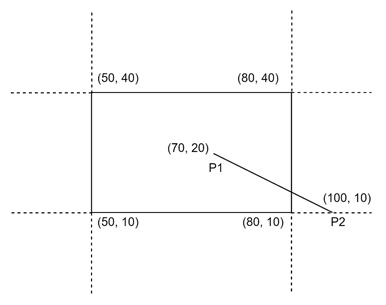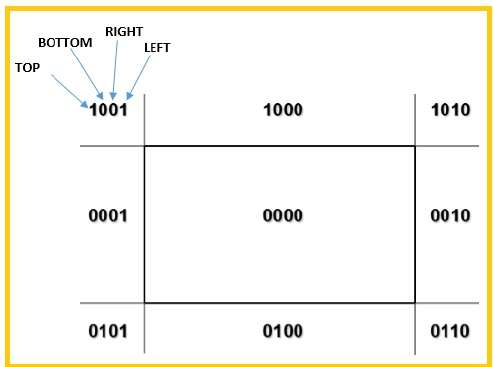# Line Clipping in computer graphics

## Line Clipping

The concept of line clipping is same as point clipping. In line clipping, we will cut the portion of line which is outside of window and keep only the portion that is inside the window.

## Cohen-Sutherland Line Clippings

This algorithm uses the clipping window as shown in the following figure. The minimum coordinate for the clipping region is \$(XW_{min,} YW_{min})\$ and the maximum coordinate for the clipping region is \$(XW_{max,} YW_{max})\$.We will use 4-bits to divide the entire region. These 4 bits represent the Top, Bottom, Right, and Left of the region as shown in the following figure. Here, the TOP and LEFT bit is set to 1 because it is the TOP-LEFT corner.There are 3 possibilities for the line −

• Line can be completely inside the window (This line should be accepted).
• Line can be completely outside of the window (This line will be completely removed from the region).
• Line can be partially inside the window (We will find intersection point and draw only that portion of line that is inside region).

### Algorithm

Step 1 − Assign a region code for each endpoints.

Step 2 − If both endpoints have a region code 0000 then accept this line.

Step 3 − Else, perform the logical ANDoperation for both region codes.

Step 3.1 − If the result is not 0000, then reject the line.

Step 3.2 − Else you need clipping.

Step 3.2.1 − Choose an endpoint of the line that is outside the window.

Step 3.2.2 − Find the intersection point at the window boundary (base on region code).

Step 3.2.3 − Replace endpoint with the intersection point and update the region code.

Step 3.2.4 − Repeat step 2 until we find a clipped line either trivially accepted or trivially rejected.

Step 4 − Repeat step 1 for other lines.

## Cyrus-Beck Line Clipping Algorithm

This algorithm is more efficient than Cohen-Sutherland algorithm. It employs parametric line representation and simple dot products.Parametric equation of line is −

```P0P1:P(t) = P0 + t(P1 - P0)
```

Let Ni be the outward normal edge Ei. Now pick any arbitrary point PEi on edge Ei then the dot product Ni.[P(t) – PEi] determines whether the point P(t) is “inside the clip edge” or “outside” the clip edge or “on” the clip edge.

The point P(t) is inside if Ni.[P(t) – PEi] < 0

The point P(t) is outside if Ni.[P(t) – PEi] > 0

The point P(t) is on the edge if Ni.[P(t) – PEi] = 0 (Intersection point)

Ni.[P(t) – PEi] = 0

Ni.[ P0 + t(P1 – P0) – PEi] = 0 (Replacing P(t) with P0 + t(P1 – P0))

Ni.[P0 – PEi] + Ni.t[P1 – P0] = 0

Ni.[P0 – PEi] + Ni∙tD = 0 (substituting D for [P1 – P0])

Ni.[P0 – PEi] = – Ni∙tD

The equation for t becomes,

\$\$t = \tfrac{N_{i}.[P_{o} – P_{Ei}]}{{- N_{i}.D}}\$\$

It is valid for the following conditions −

• Ni ≠ 0 (error cannot happen)
• D ≠ 0 (P1 ≠ P0)
• Ni∙D ≠ 0 (P0P1 not parallel to Ei)